# 图神经网络全面综述

This survey considers GNNs as all deep learning aproaches for graph data.

## Notations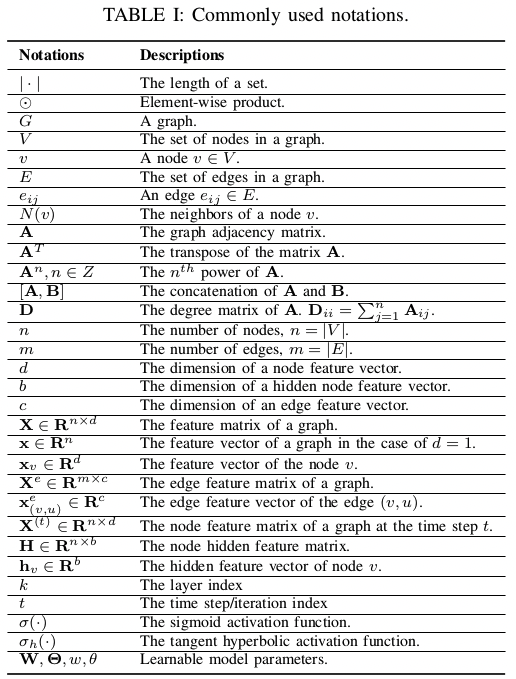## 本文调研的 RecGNN 和 ConvGNN 模型一览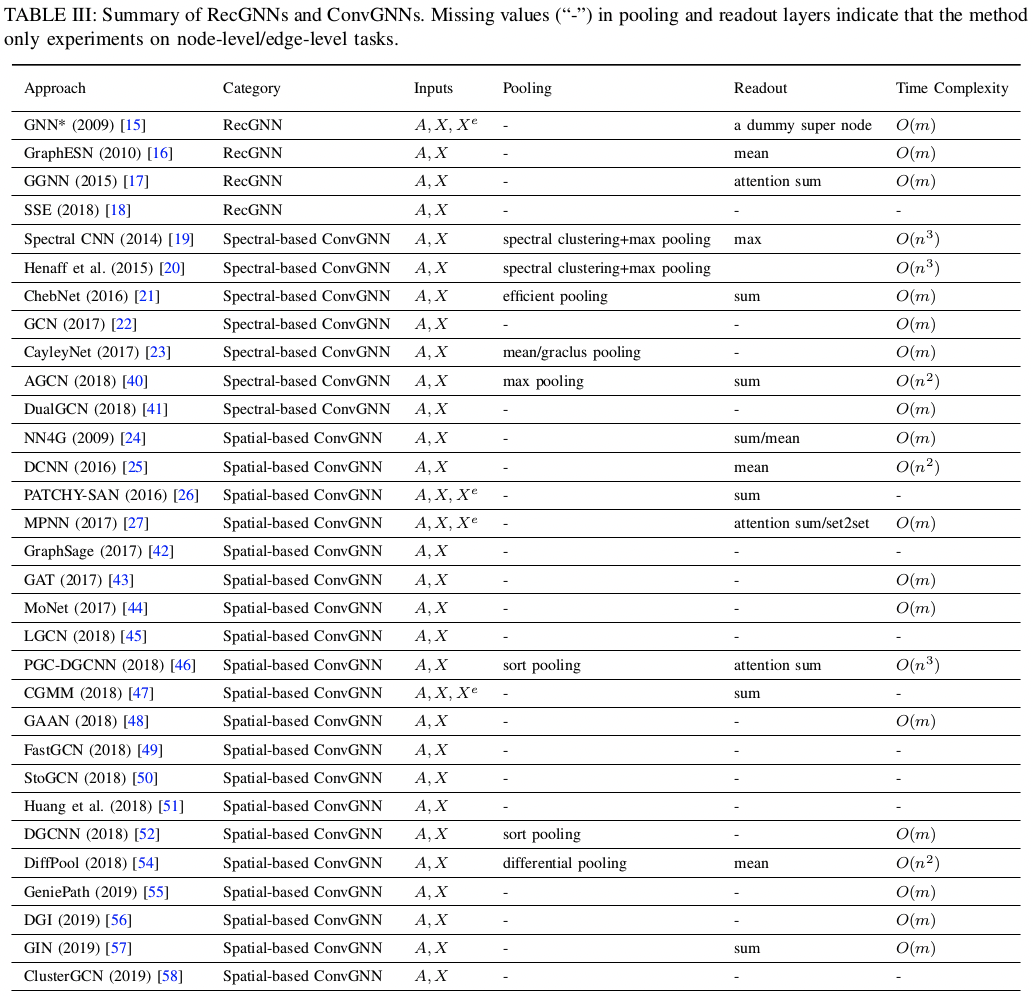## Recurrent Graph Neural Networks, RecGNNs

1. Graph Neural Network (GNN)

• $h_v^{(t)} = \sum\limits_{u \in N(v)} f_\theta(x_v, x^e_{(v, u)}, x_u, h_u^{(t-1)})$
• $f_\theta$ 须使两点映射后距离更近，才能保证收敛。如果 $f_\theta$ 是神经网络，则要有对 Jacobian matrix 的正则项。
• Graph Echo State Network (GraphESN) 改进了训练性能
2. Gated Graph Neural Network (GGNN)

• $h_v^{(t)} = \text{GRU}(h_v^{(t-1)}, \sum\limits_{u \in N(v)} W_\theta h_u^{(t-1)})$
• Gated Recurrent Unit (GRU)

• $h_v^{(t)} = (1 - \alpha)h_v^{(t-1)} + \alpha W_{\theta_1} \sigma (W_{\theta_2} [x_v, \sum\limits_{u \in N(v)} [h_u^{(t-1)}, x_u]])$
• 随机采样小批量节点异步更新状态与计算梯度，因此可扩展到大规模图中
• 但没证明收敛性

## Convolutional Graph Neural Networks, ConvGNNs

### Spectral-based ConvGNNs

Spectral-based 方法主要就是学习这个滤波器 $g = g_\theta$，不同方法对其引入了不同的归纳偏置（Inductive Biases）。

1. Spectral CNN

• $H_j^{(l)} = \sigma(\sum\limits_{i=1}^{d_{l-1}} H_i^{(l-1)} * g_{i,j;\theta}^{(l)}), j = 1, 2, \cdots, d_k$
• $H^{(0)} = X$，$H_i$ 是 H 的第 i 列，代表一个 channel
• 或记为 $H^{(l)} = \sigma(H^{(l-1)} * W_\theta^{(l)})$，其中 H 为向量、W 为矩阵，但它们的元素都为 n 维向量，元素相乘为卷积
2. Chebyshev Spectral CNN (ChebNet)

• 将特征值压缩到 [-1, 1]：$\tilde{\Lambda} = 2 \Lambda / \lambda_{\max} - I$
• 用 $\Lambda$ 的 K 次多项式逼近 $g_\theta$ 并表示为 Chebyshev Polynomials $T_n(x) = \cos(n \arccos x)$ 的加权和：$$\begin{cases} g_\theta = \sum\limits_{i=0}^{K} \theta_i T_i(\tilde{\Lambda}) \\ T_i(x) = 2xT_{i-1}(x) - T_{i-2}(x) \\ T_0(x) = 1, T_1(x) = x \end{cases}$$
• 于是 $$x * g_\theta = U (\sum\limits_{i=0}^{K} \theta_i T_i(\tilde{\Lambda})) U^T x = \sum\limits_{i=0}^{K} \theta_i T_i(2L / \lambda_{\max} - I) x$$
• 可独立于图的尺度提取局部特征 [^TODO: 不太理解，可能跟切比雪夫多项式的特性有关，比如不同 i 代表不同的尺度]
• CayleyNet 引入 Cayley Polynomials 定义 $$x * g = c_0 x + 2 \text{Re}{\sum\limits_{j=1}^{r} c_j(hL - iI)^j(hL + iI)^{-j}x}$$ ChebNet 算它的一个特殊情况
1. Graph Convolutional Network (GCN)

• ChebNet 中令 $K = 1, \lambda_{\max} = 2, \theta_0 = -\theta_1$ 就得 GCN $$x * g_\theta = \theta(I + D^{-\frac{1}{2}} A D^{-\frac{1}{2}})x \approx \theta((D + I)^{-\frac{1}{2}} (A + I) (D + I)^{-\frac{1}{2}})x = \theta(\tilde{D}^{-\frac12} \tilde{A} \tilde{D}^{-\frac12})x$$ 其中近似部分是为了通过平滑解决数值稳定性问题
• 相邻层隐状态之间的 channel 全连接，写为 $$H^{(l+1)} = \sigma (\tilde{D}^{-\frac12} \tilde{A} \tilde{D}^{-\frac12} H^{(l)} W_\theta^{(l)})$$
• 因为 $\tilde{D}^{-\frac12} \tilde{A} \tilde{D}^{-\frac12}$ 只在图中有边的对应位置有值，所以这个卷积操作又可以看作是在聚合相邻节点的信息，故 GCN 同时也算 Spatial-based 的方法
2. Adaptive Graph Convolutional Network (AGCN)

• 邻接矩阵中的值不只是 0 和 1，而是经一个可学习的距离函数由两节点的特征得到的输出值
3. Dual Graph Convolutional Network (DGCN)

• 在图中随机游走后生成衡量节点共现程度的 PPMI 矩阵（见 Information Theory）记为 B，也做卷积后与 GCN 的卷积混合 $$H^{(l+1)} = \sigma (\tilde{D}^{-\frac12} \tilde{A} \tilde{D}^{-\frac12} H^{(l)} W_{\theta_1}^{(l)} + BH^{(l)}W_{\theta_2}^{(l)})$$ [^TODO: 不太清楚是每一层都混合还是到最后一层再混合，综述没说清楚，要看原论文。但倾向是前者]
• 因为 A 带了局部的信息，B 带了全局的信息，所以最终的表示不需要堆叠起多层结果来得到

Spectrul-based 方法的缺点

1. 邻接矩阵的轻微变化会改变特征值与特征向量，使得整个表示受影响
2. 学到的滤波器因此严重依赖于邻接矩阵，难以运用到其它结构的图中
3. 特征值分解得到 U 的复杂度为 O($n^3$)，效率低而且不能小批量训练

### Spatial-based ConvGNNs

1. Neural Network for Graphs (NN4G)

• 聚合自身属性和周围节点的各层堆叠隐状态 $$H^{(k)} = \sigma(XW^{(k)} + \sum\limits_{i=1}^{k-1} AH^{(k-1)}\Theta^{(k)})$$
• Contextual Graph Markov Model (CGMM): NN4G fork with probabilistic interpretability
2. Diffusion Convolutional Neural Network (DCNN)

• $H^{(k)} = \sigma(W^{(k)} \otimes P^k X)$，其中 $P = D^{-1}A$ 是转移概率矩阵
• 输出各层 H 的拼接
• Difussion Graph Convolution (DGC): $H = \sum\limits_{k=0}^{K} \sigma(P^k X W^{(k)})$
• PGC-DGCNN 认为转移矩阵衰减太快，所以定义 $S^{(j)}{u,v}$ = bool(u, v 有长为 j 的最短路径)，隐状态为 $\sigma((\sum_l S^{(j)}{i,l})^{-1} S^{(j)} H^{(k-1)} W^{(j,k)}), j = 0, \cdots, r$ 的拼接
3. Partition Graph Convolution (PGC)

• 泛化了 PGC-DGCNN 中分组的思想，将邻接节点按一定原则分为 Q 组分别做不激活的 GCN 后加起来，作为新的状态
4. Message Passing Neural Network (MPNN)

• 应该说是一种（消息传递的）框架
• $h_v^{(k)} = U_k (h_v^{(k-1)}, \sum\limits_{u \in N(v)} M_k (h_v^{(k-1)}, h_u^{(k-1)}, x_{vu}^e))$
5. Graph Isomorphism Network (GIN)

• 基于 MPNN 的网络无法通过学到的表示分辨不同的结构
• $h_v^{(k)} = \text{MLP}((1 + \epsilon^{(k)}) h_v^{(k-1)} + \sum\limits_{u \in N(v)} h_u^{(k-1)})$
6. GraphSage

• 通常的网络在上规模后效率都成问题，GraphSage 只采样部分邻居节点，是一个工业可用的模型
7. Graph Attention Network (GAT)

• 使用注意力网络为节点之间的连接分配权重，在节点分类任务上效果远超 GraphSage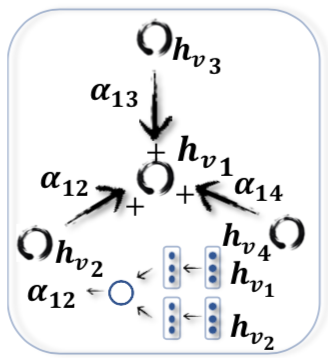• 用了多头注意力，但权重相等
• Gated Attention Network (GaAN) 又加了个自注意力给每个头加权
8. Mixture Model Network (MoNet)

• 把 GNN 的方法用到了流形的深度学习上，将流形中的点视为图的节点，用相对坐标来决定边的权重
9. PATCHY-SAN

• 每个节点都按一定规则为邻居排序、之后截取前 q 个保持边，这样把图结构转换为网格结构
10. Large-scale Graph Convolutional Network (LGCN)

• 对各节点所有邻居的每一种特征进行特征内的排序，保持前 q 个值，形成的特征矩阵作为中心节点的表示来源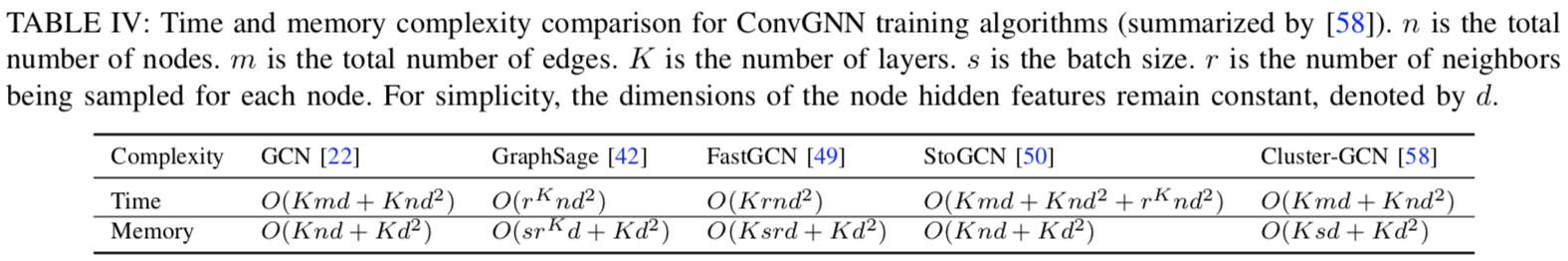1. GraphSage 内存较省，但时空复杂度都随 r、K 指数级上升

2. Fast Learning with Graph Convolutional Network (FastGCN)

• 每层只采样固定数量的节点
• 将卷积层视为在概率度量上的嵌入函数的积分形式[^TODO: 不懂，需要看原论文]，因此训练中用到蒙特卡罗估计和变分
• Huang et al. 又提出一种逐层采样方法，提升了 FastGCN 的准确率
3. Stochastic Training of Graph Convolutional Networks (StoGCN)

• 通过节点的历史表示极大地减小了感知域，即使每个节点只采样两个邻居也能有好的表现
• 在这几个方法里时间复杂度最高，内存瓶颈也没解决
4. Cluster-GCN 通过图聚类算法在图中采样出子图，并在子图上做卷积

• 内存最低

## Graph Autoencoders, GAE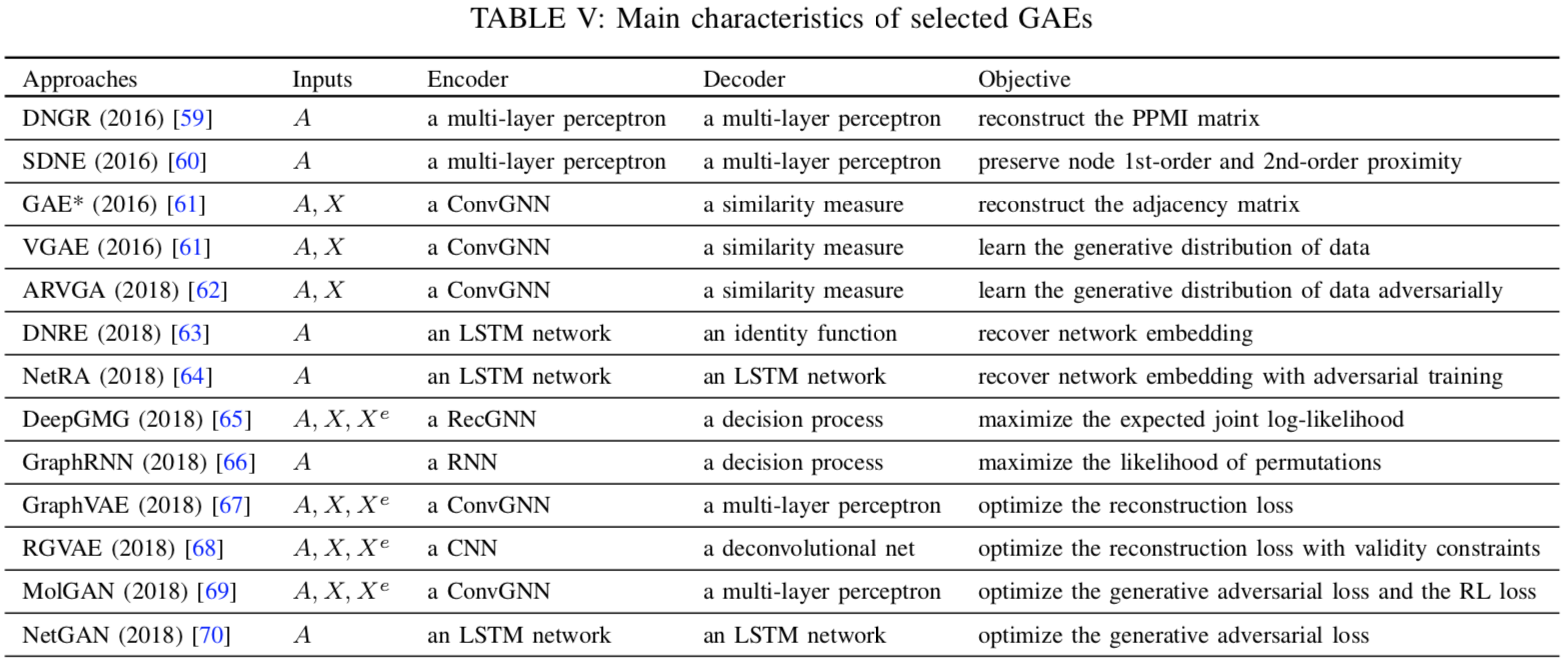### Network Embedding

1. Deep Neural Network for Graph (DNGR)

• 通过多层感知机重建 PPMI 矩阵
2. Structural Deep Network Embedding (SDNE)

• 第一个损失函数最小化节点的嵌入及其邻居的嵌入的距离
• 第二个损失函数最小化节点表示及其重建表示之间的距离
3. Graph Autoencoder (GAE)

• 用图卷积层同时考虑邻接矩阵与节点特征
• 重建邻接矩阵 $\hat A_{v,u} = \text{dec}(z_v, z_u) = \sigma (z_v^T z_u)$，其中 z 是节点的嵌入
• GraphSage 中对于无监督任务（就是做嵌入）也是用的与逆采样的交叉熵
4. Variational Graph Autoencoder (VGAE)

• 自编码器能力过强通常会过拟合，所以 VGAE 优化 ELBO
5. Adversarially Regularized Variational Graph Autoencoder (ARVGA)

• 引入 GAN 的训练方式
6. Deep Recursive Network Embedding (DRNE)

• 上面都是基于链接预测来做嵌入，但图中链接通常都是稀疏的。所以这方法随机采节点的邻居训练一个 LSTM，把图的训练转化为对序列的训练，来回避链接稀疏的问题
7. Network Representations with Adversarially Regularized Autoencoders (NetRa)

1. DeepGMG
2. GraphRNN

1. GraphVAE
2. RGVAE
3. MolGAN
4. NetGAN

## Spatial-Temporal Graph Neural Networks, STGNN

RNN-based 的方法通常是在 RNN 后接 GCN，即 $$H^{(t)} = \sigma (\text{Gconv}(X^{(t)}, A; W) + \text{Gconv}(H^{(t-1)}, A; U) + b)$$ 比如：

• Graph Convolutional Recurrent Network (GCRN) 是 LSTM + ChebNet
• Diffusion Convolutional Recurrent Neural Network (DCRNN) 是 GRU + DGC
• 不过另外还用了个编解码器架构预测 K 步后节点的值
• Structural-RNN 则是 edge-RNN 后接 node-RNN

CNN-based 的方法能并行、内存更低、梯度更稳定，比如 STGCN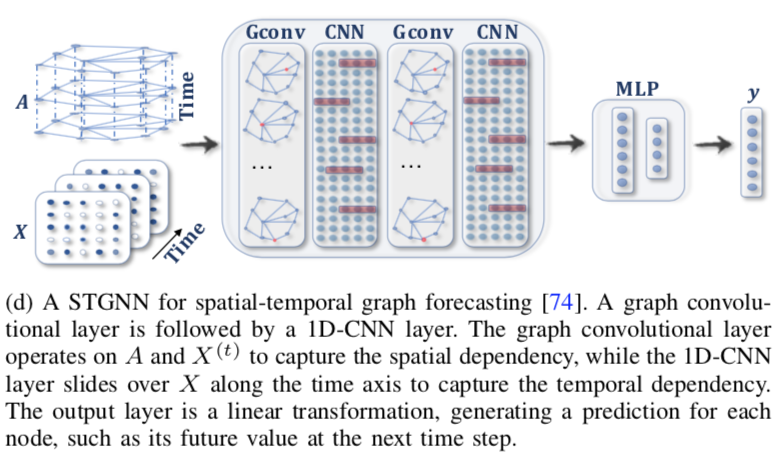Graph WaveNet 提出既然有了各节点在不同时刻的值，就可以据此学到节点间的关系，即 $A = \text{SoftMax}(\text{ReLU}(E_1E_2^T))$，其中 E 是节点的表示。这样就不需要图本身的邻接矩阵。

GaAN 和 ASTGCN 又引入了注意力机制。